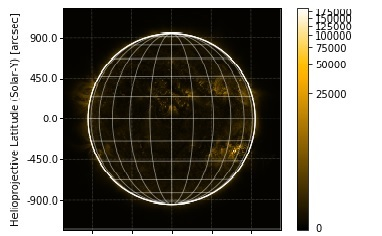# Plotting solar image in Python

In Python provides SunPy package for create solar image. In this package has different files which are solar data of proton/electron fluxes from various solar observatory and solar labs.

Using pip install sunpy command, we can install sunpy package.

Here we plot a sample AIA image. AIA is Atmospheric Imaging Assembly. This is another instrument board of the SDO.

Here we use sunpy.Map() function to create a map from one of the supported data products.

## Example code

import sunpy.map
import matplotlib.pyplot as plt
import sunpy.data.sample
my_aia = sunpy.map.Map(sunpy.data.sample.AIA_171_IMAGE)
fig = plt.figure()
ax = plt.subplot(111, projection=my_aia)
my_aia.plot()
my_aia.draw_limb()
my_aia.draw_grid()
my_aia.draw_limb()
plt.colorbar()
plt.show()


## Output